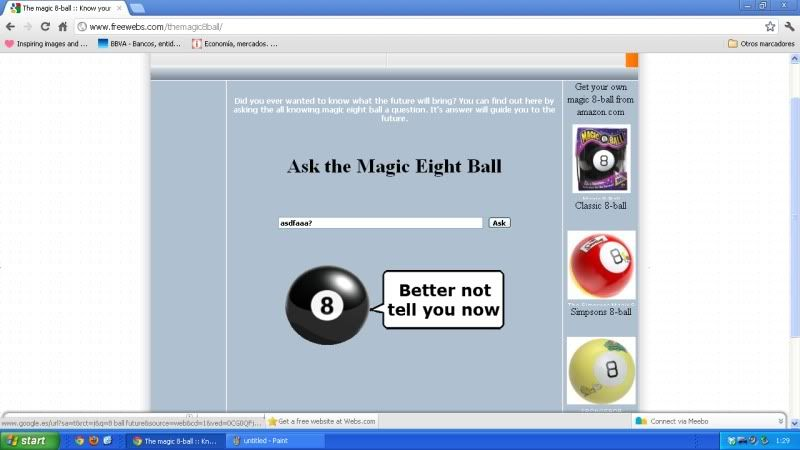#the crazy monty hall problem• ### General discussion

•isnt this like seeing the future?

is it correct?

re:
'this is the door of 3 behind behind which it is the prize
a=math.GetRandomNumber(3)
'this is the door the host discarded the previous week
b= Math.GetRandomNumber(3)
'this is the door number you pick without having wathced the show the previous week
'and not knowing a door has been discarded
c= Math.GetRandomNumber(3)
'this is in case you pick the discraded by the host door by
'which you would be sent to sleep and start over next week
If b=c Then
Goto re
EndIf
'there are two left doors so its a 50% chance

If a=c Then
'If you get the right door right you win one coin
bob=bob+1
Else
'if you get the wrong door you lose one coin
bob=bob-1
EndIf
'remember its a 50% chance there being two door, if you win you win one if you lose you lose one
'now you can run the program and check how bad you lose for not swithcing door
TextWindow.WriteLine(bob)
Goto re

another:

re:
'this is the door of 3 behind behind which it is the prize
a=math.GetRandomNumber(3)
re1:
'this is the door the host discarded the previous week
b= 3
'this is the door number you pick without having wathced the show the previous week
'and not knowing a door has been discarded
c= 1
'this is in case you pick the discraded by the host door by
'which you would be sent to sleep and start over next week
If b=c Then
Goto re1
EndIf
'there are two left doors so its a 50% chance

If a=c Then
'If you get the right door right you win one coin
bob=bob+1
Else
'if you get the wrong door you lose one coin
bob=bob-1
EndIf
'remember its a 50% chance there being two door, if you win you win one if you lose you lose one
'now you can run the program and check how bad you lose for not swithcing door
TextWindow.WriteLine(bob)
Goto re

Tuesday, May 8, 2012 9:48 PM

### All replies

•now something crazy on the future teller 8 ball, IT WORKS

the key for this is that in a 2 choice option you add a virtual 3rd option

so you trow a 3 sides dice and every time it appears the virtual option you say plz ask another time

then applying the monty hall thing you have a 66% chance to be right following the 8 ball where if you didnt you would have just a 50%

seems the truth its been always out there:another identical thing between my program and the magic 8ball:

from the wiki:

To use the ball, it must be held with the window initially facing down. After "asking the ball" a yes-no question (and shaking the ball), the user then TURNS the ball so that the window faces up,

Tuesday, May 8, 2012 11:39 PM
•For me this shows a bunch of negative numbers...
Wednesday, May 9, 2012 12:40 AM
•For me this shows a bunch of negative numbers...

yes thats the money you lose in a hteoretically 50% chance game in which if you win you win one and if you lose you lose one as well

it should remain in zero but as you dont switch you lose 2/3 of times in a 1/2 chance game

watch this:

edit:

its wrong, the logic is wrong its posible with this program the prize and the discarded door its the same

Wednesday, May 9, 2012 1:47 AM
•here the program corrected i think its right now:

re:
'this is the door of 3 behind behind which it is the prize
a=math.GetRandomNumber(3)
'this is the door the host discarded the previous week, you have bad memory but you remember
'it was not discarded door number 3
re1:
b= Math.GetRandomNumber(2)

'this is so the discarded door is different than the price
If a=b Then
Goto re1
EndIf
'this is the door number you pick without being very sure which was the discraded door
'as you know for sure the 3 wasnt discarded you decide to always pick door number 3 initially
c= 3
'there are two left doors so its a 50% chance
If a<>c Then
'If you get the right door right you win one coin
bob=bob+1
Else
'if you get the wrong door you lose one coin
bob=bob-1
EndIf
'remember its a 50% chance there being two door, if you win you win one if you lose you lose one
'now you can run the program and check how much you win by switching the pick